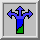# The One-Dimensional Random Walk1-D Random Walk Java Applet

Random movement is important for understanding the microscopic world in Nature, because atoms and molecules move randomly. How can we describe the random motion of molecules in, say, a gas? Molecules are too small to see, so to help us think concretely we replace a molecule with something we can see: a wandering ant. If a wandering ant starts at a lamp post and takes steps of equal length along the street, how far will it be from the lamp post after a certain number, say N, steps? Though this question is seemingly trivial, it poses one of the most basic problems in statistical science.First, let's take a look at a simple coin-flipping experiment. Suppose you flip ten coins, count the number of heads, and plot this number on a histogram. What do you suppose the histogram would look like after, say, 100 trials? Do you believe it will look something like the histogram in Figure 1? What do you suppose will happen if we were to make 1,000 trials? 10,000 trials? What you are forming is a bell-shaped curve (also called a normal or Guassian distribution).

What does Figure 1 tell us? Randomness (coin-flipping) leads to some sort of predictable outcome (the bell-shaped curve).

This Applet relates random coin-flipping to random motion. It is easiest to visualize random motion (random walk) along one line, that is, in one dimension. Call `x` the position of the ant (i.e., walker) on a one-dimensional line. Locate the origin, that is `x = 0`, at the lamp post. Then let each ``step'' of the ant - right or left along the line - be of equal length. One way to picture this is to use one row of a checkerboard or an enlarged photocopy of Figure 2.Figure 2: Diagram of a wandering ant. How many steps do you think he has already taken if his starting-point was at the ``Lamp post''?

Choose the direction of the step the ant will take by flipping a penny: (1) If it is a head, the ant steps right and `x` increases by one. (2) If it is a tail, the ant steps left and `x` decreases by one. A head or tail is equally likely; therefore it is equally probable that the ant steps right or left.

Do this activity with a partner. Use a silver-colored coin (nickel, dime, or quarter) to represent the position of the ant. To begin, put the ``ant'' in a center cell (the position of the lamp post). The ant steps from one cell to the next, right or left randomly, depending on whether the penny comes up heads or tails, respectively.

• Flip a penny ten times and move your ``ant'' accordingly.
• After ten steps, report the final position of the ant, and whether it is to the right or to the left of the lamp post.
• Again, the tally keeper puts a big `X` on a bar graph of the final position on the blackboard, as in Figure 1, above.

What is so important about the graph? Science is about making predictions, often based on measuring some quantities. You will find that the measure of the mean squared distance is one of the most important and powerful measures of a random walk.

How far from the origin does the wandering ant end up after some number (`N`) of steps? What do you guess: after 10 steps is the walker more likely to be to the right or to the left of its starting point? If another ant takes 10 steps from the starting point, then another ant, then another ant, what do you expect their average final position to be after 10 steps?

What does theory have to say about the value of the average position of many random walkers? Answer: The value of the average position is zero, the position of the starting point (the lamp post)! How can this be? One word gives the reason: Symmetry! In this case symmetry means that moving right is just as likely as moving left. After any fixed number of steps, the walker is equally likely to be to the right of the starting point (positive displacement) as to the left (negative displacement). Moreover, for a given number of steps, the distance `x` from the starting point is likely to have the same value, whether displacement is to the right (positive `x`) or to the left (negative `x`). In brief, averaged over many millions of trials, the positive displacements cancel the negative displacements. Therefore we expect the average of many trials to be zero; the average position of the walker is at the starting point. The average position may be zero, but the spread of positions is not zero. How can this spread be described? There are various ways to measure this spread. Scientists often use the symbol `x` to represent displacement along a line, `x**2` to represent the square of this displacement, and a bracket "< >" to represent average value. Let's measure the spread by looking at the average squared distance or < x**2 > as a function of the step number `N`. The plot in the Applet measures this quantity. Can you draw any conclusions from it? Hint: Try and extract an equation from the plot relating the mean squared distance to the step number.

Now that you've had a chance to experiment, can you answer these questions?

• Does changing the number of steps the walker takes effect the relation between "mean squared distance" and "step number"? Does it change the "average distance" from the origin?

• Why would we care about being able to relate "mean squared distance" and "step number"? What does this tell us? Hint: think in terms of "predicting".

• Can you think of examples from nature where particles may move around in a random way?